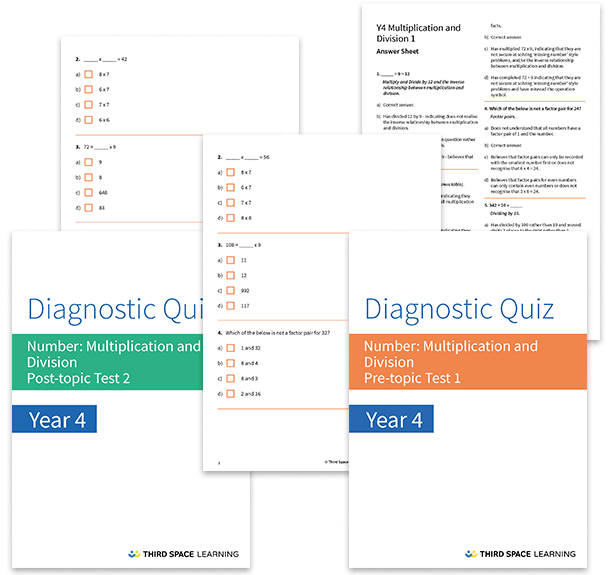# Multiplication and Division Diagnostic Assessments Year 4

This pack of resources contains two diagnostic assessments for multiplication and division, one pre and one post topic assessment. Each test contains 23 multiple choice questions with detailed answers that not only indicate the correct answer but also explain the distractor answers. You can use these tests to help you diagnose specific misconceptions and learning gaps before starting a block of learning and after the block has been completed.

These tests cover:

• Times table facts (multiplication and division) for the 1 to 12 times tables
• Multiplying and dividing by 10 or 100
• Multiplying by 0
• Multiplying by 25
• Mental multiplication
• Multiplying a three-digit number by a one-digit number
• Scaling calculations
• Factor pairs

Year 4

Multiplication, Division